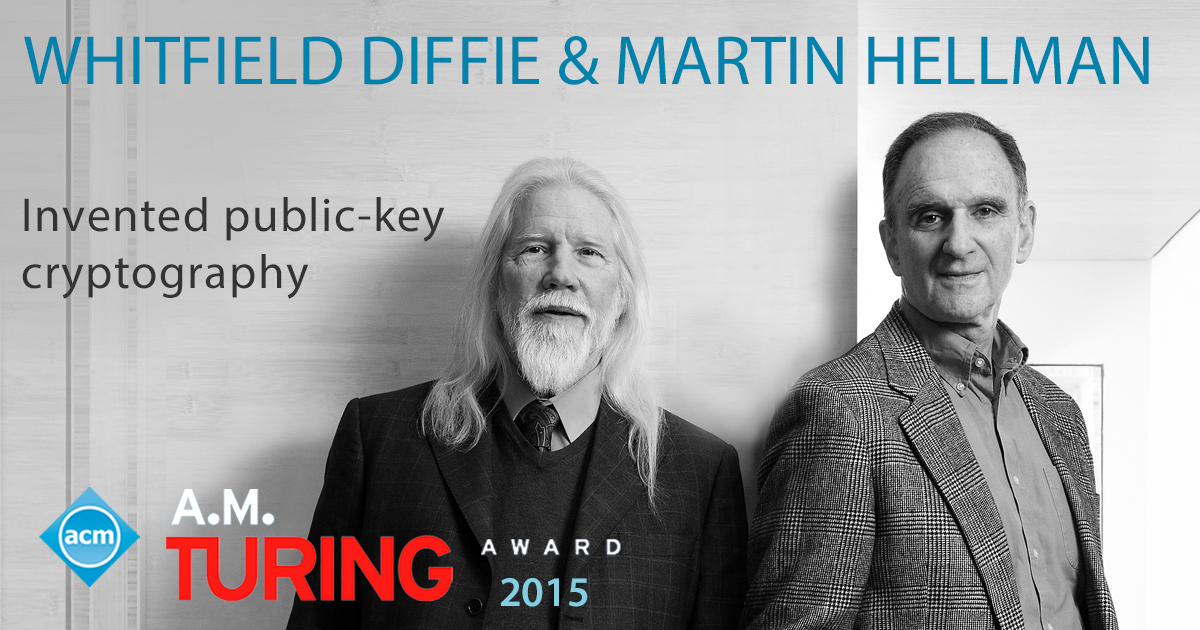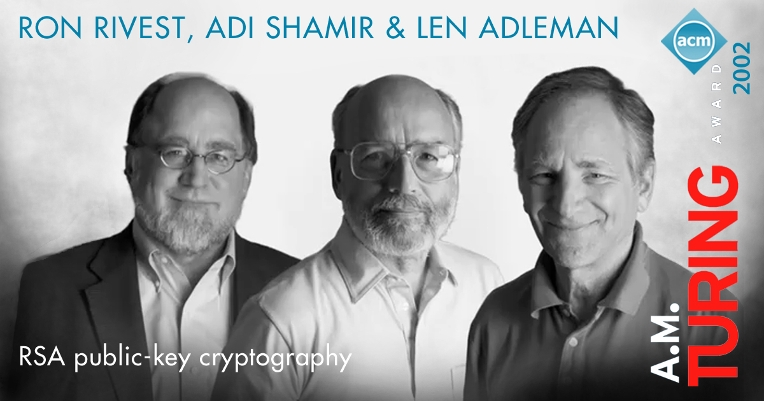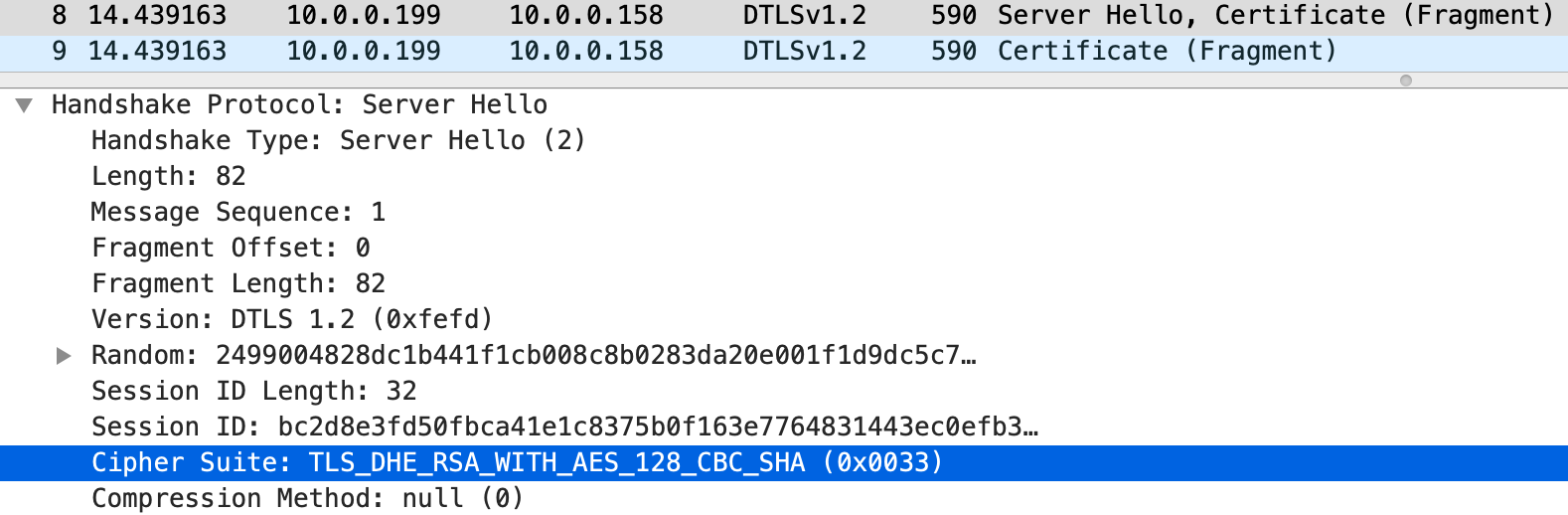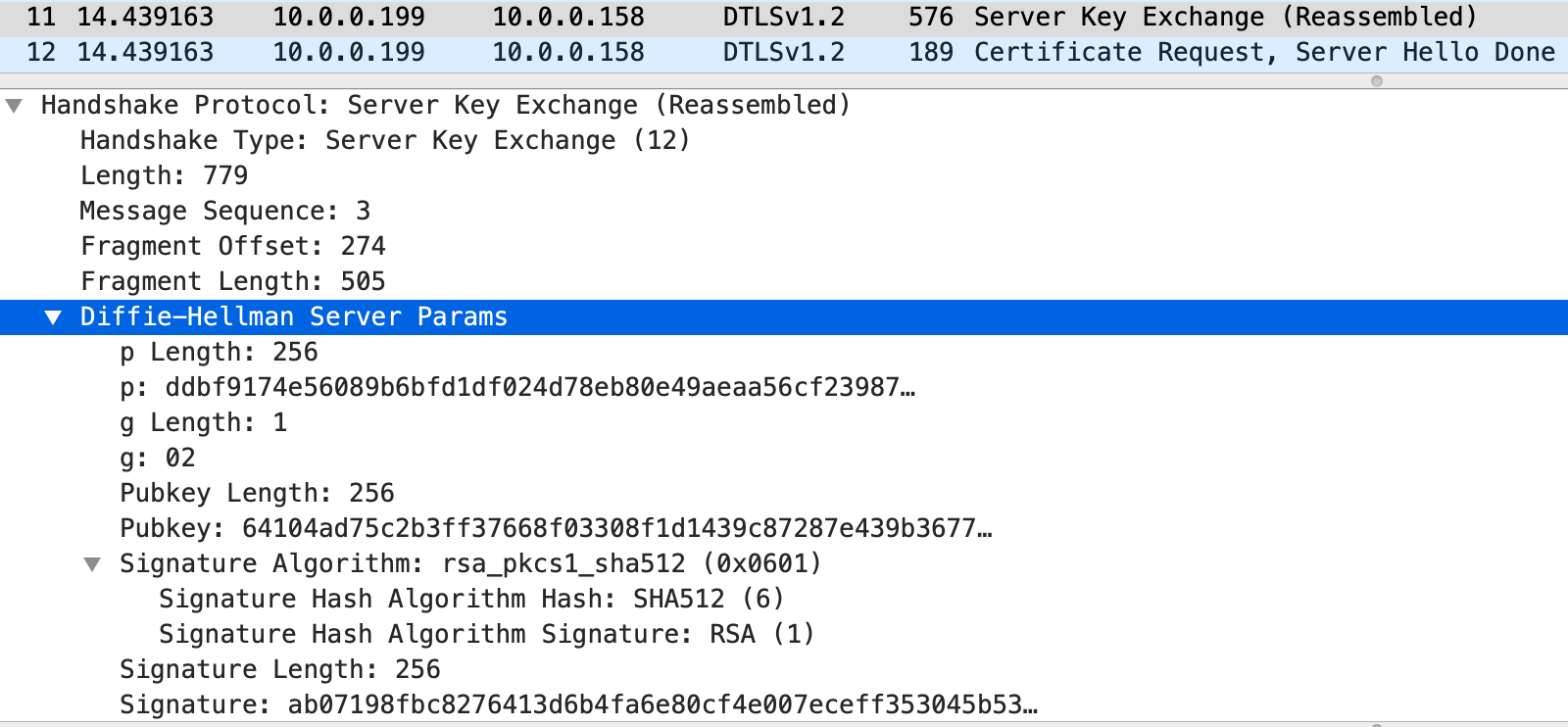A cryptographic system should be secure even if everything about the system, except the key, is public knowledge.
Auguste Kerckhoffs（奥古斯特·柯克霍夫，荷兰语言学家和密码学家，提出密码学的“柯克霍夫原则”）

## 迪菲—赫尔曼密钥交换

1. 爱丽丝选定一个素数 $$p=71$$，再选定整数模 $$p$$ 乘法群的一个原根 $$g=7$$
2. 爱丽丝选定一个小于 $$p$$ 的随机数 $$a=17$$，计算 $$A=g^a\;mod\;p=7^{17}\;mod\;71 = 62$$
3. 爱丽丝将 $$(p,g,A)$$ 一起发给鲍勃
4. 鲍勃也选定一个小于 $$p$$ 的随机数 $$b=39$$，计算 $$B=g^b\;mod\;p=7^{39}\;mod\;71 = 13$$
5. 鲍勃将 $$B$$ 发回给爱丽丝
6. 爱丽丝计算 $$s=B^a\;mod\;p=13^{17}\;mod\;71 = 42$$
7. 鲍勃计算 $$s=A^b\;mod\;p=62^{39}\;mod\;71 = 42$$

$A^b\;mod\;p=g^{ab}\;mod\;p=g^{ba}\;mod\;p=B^a\;mod\;p$

• $$A=g^a\;mod\;p\Rightarrow \color{fuchsia}{a = log_g A\;mod\;p}$$
• $$B=g^b\;mod\;p\Rightarrow \color{fuchsia}{b = log_g B\;mod\;p}$$## RSA加密算法

RSA 是一种公钥加密算法，以此为核心技术构成的同名公钥加密系统，被广泛应用于保密数据传输。今天，互联网的全面发展已经在社会的各个层面为大众提供极大便利。不论你是在网上冲浪、游戏、娱乐、购物、还是与亲友即时通讯、管理银行账户、投资买卖金融证券，或者只是简单地收发电子邮件，都有 RSA 在幕后运行保障你的隐私和数据安全。

RSA 实际上是三个人姓氏的缩写，他们是美国密码学家罗纳德·李维斯特 (Ronald Rivest)、以色列密码学家阿迪·沙米尔 (Adi Shamir) 和美国计算机科学家伦纳德·阿德曼 (Leonard Max Adleman) 。1977年，李维斯特、沙米尔和阿德曼三人在麻省理工学院 (MIT) 合作共同发明了 RSA 加密算法。算法最先发布在MIT的公开技术报告里，后来整理发表在1978年二月的 ACM 通讯 杂志，标题为《一种获取数字签名和公钥密码系统的方法》。

RSA 的基本思想是使用者创建由一个公钥和一个私钥组成的密钥对。公钥自由发布，私钥必须秘密保存。任何人都可以用公钥来加密消息，而生成的密文只有私钥持有者才能解读。另一方面，以私钥加密的信息，公钥都可以解开。由于我们假定私钥只是特定对象才能持有，所以使用私钥加密相当于生成数字签名，用公钥解密等效于验证签名。

RSA 加密算法包括四步操作过程：密钥生成、密钥分配、加密和解密。下面也举一个简单而具体的例子来说明：

1. 爱丽丝随机选择两个素数 $$p=127$$$$q=5867$$，计算 $$N=pq=745109$$
2. 爱丽丝计算 $$N$$卡迈克尔函数 $$\lambda(N)=\lambda(745109)=52794$$
• $$p$$$$q$$ 都为素数时，通常 $$\lambda(pq)=lcm(p − 1, q − 1)$$
• $$lcm$$ 是求最小公倍数的函数，可以用欧几里得算法得出
• $$lcm(126,5866)=52794$$
3. 爱丽丝选择一个小于 $$\lambda(N)$$ 且与之互素的数 $$e=5$$，并求得 $$e$$ 关于 $$\lambda(N)$$模逆元 $$d\equiv e^{-1}\pmod {\lambda(N)}$$，得到 $$d=10559$$
• 模逆元的定义是，找到 $$d$$ 使得 $$(d⋅e)\;mod\;\lambda(N)=1$$
• $$d=10559\equiv 5^{-1}\pmod {52794}$$
4. $$\pmb{(N,e)}$$ 就是爱丽丝的公钥$$\pmb{(N,d)}$$ 是她的私钥
• 爱丽丝将她的公钥 $$(745109,5)$$ 发给鲍勃
• 爱丽丝密藏她的私钥 $$(745109,10559)$$
• 爱丽丝销毁所有 $$p,q,\lambda(N)$$ 的记录
5. 当鲍勃想给爱丽丝送一个消息 $$M$$ 时，先按照双方约定好的编码格式将 $$M$$ 转化为一个或多个小于 $$N$$ 的正整数 $$m$$，然后使用爱丽丝的公钥逐个计算出密文 $$c$$。计算公式是 $$\pmb{c\equiv m^e\pmod N}$$
• 假定 $$M$$ 为“CACC 9678”，编码格式是空格为0、a-z/A-Z（忽略大小写）为1-26、0-9为27-36
• 转化后得到正整数串 “030103 030036 333435”，注意每个都小于745109
• 加密后的密文整数串 “184539 741303 358095”
• $$184539 \equiv 30103^5\pmod {745109}$$
• $$741303 \equiv 30036^5\pmod {745109}$$
• $$358095 \equiv 333435^5\pmod {745109}$$
6. 爱丽丝收到密文整数串后，使用自己的私钥逐个计算出明文 $$m$$，计算公式是 $$\pmb{m\equiv c^d\pmod N}$$
• $$30103 \equiv 184539^{10559}\pmod {745109}$$
• $$30036 \equiv 741303^{10559}\pmod {745109}$$
• $$333435 \equiv 358095^{10559}\pmod {745109}$$

$52794s+5t=gcd(5, 52794)=1$

0 $$52794$$ $$1$$ $$0$$
1 $$5$$ $$0$$ $$1$$
2 $$52794 \div5 = 10558$$ $$4$$ $$1 - 10558\times 0 = 1$$ $$0 - 10558\times 1 = -10558$$
3 $$5 \div4 = 1$$ $$1$$ $$0-1\times1 = -1$$ $$1 - 1\times (-10558) = \bf10559$$

$\Rightarrow m^{ed} = m^{(1+h\lambda(N))} = \color{fuchsia}{m(m^{\lambda(N)})^h \equiv m(1)^h}\equiv m\pmod N$

RSA 公钥加密算法，是三位密码学家和计算机科学家的天才创造。它的发明是公钥密码技术新的里程碑，也成为现代互联网安全通信的基石。李维斯特、沙米尔和阿德曼的杰出贡献，为他们赢得了2002 年的 ACM 图灵奖，比迪菲和赫尔曼早了足足13年！## 区别与联系

RSA 公钥加密算法在实际应用中存在一个问题，即它没有前向保密的功能。前向保密 (Forward Secrecy)，有时也被称为完全前向保密 (Perfect Forward Secrecy)，是保密通信协议的一种安全属性，指的是长期使用的主密钥泄漏不会导致过去的会话信息泄漏。如果系统具有前向保密性，就可以保护在私钥泄露时历史通信纪录的安全。设想一下这样的情况，虽然伊芙无法破解爱丽丝与鲍勃之间用 RSA 加密的消息，伊芙可以存档全部的过往消息密文。未来某一天，爱丽丝的私钥因为某种原因被泄漏，那么伊芙就可以解密所有的消息记录。

1. 爱丽丝与鲍勃双方交换经过认证的 RSA 公钥证书
2. 爱丽丝与鲍勃各自产生随机的 $$(a,b)$$ 值，用共享的迪菲-赫尔曼 $$(p,g)$$ 计算出 $$(A,B)$$
3. 爱丽丝用自己的 RSA 私钥加密 $$A$$ 生成数字签名，将之与 $$A$$ 一起发给鲍勃
4. 鲍勃用自己的 RSA 私钥加密 $$B$$ 生成数字签名，将之与 $$B$$ 一起发给爱丽丝
5. 爱丽丝用鲍勃的 RSA 公钥验证签名，确认 $$B$$ 来自鲍勃，用 $$(p,a,B)$$ 算出 $$s$$
6. 鲍勃用爱丽丝的 RSA 公钥验证签名，确认 $$A$$ 来自爱丽丝，用 $$(p,b,A)$$ 算出 $$s$$
7. 爱丽丝和鲍勃达成共享秘密，生成后续对称加密 (AES) 的会话密钥，进行保密通信

## DHE-RSA加密套件

TLS 协议采用主从式（客户机/服务器）架构模型。它的工作模式，是使用 X.509 认证和非对称加密算法对通信方做身份认证，之后交换密钥生成对称加密的会话密钥。这个会话密钥就用来加密通信双方交换的数据，保证信息的保密性和可靠性，不必担心被第三方攻击或窃听。为了标识方便，TLS 1.2 协议将使用的身份验证、密钥交换、批量加密和消息认证码算法 组合成密码套件 (Cipher Suite) 名称。每个密码套件被赋予一个双字节的编码。TLS 密码套件注册表提供了全部登记在录的密码套件命名参考表，参考表以编码值从小到大排序。

TLS 1.2 协议支持一系列组合迪菲-赫尔曼密钥交换与 RSA 公钥加密算法的密码套件。它们都以TLS_DH_RSATLS_DHE_RSA作为开头。DHE 中的 “E” 代表 “Ephemeral” (临时的)，其意义是要求每次会话都要产生随机的 $$(a,b)$$ 值。所以TLS_DHE_RSA密码套件能提供前向保密，而TLS_DH_RSA不可以，实际应用中应该优先选择前者。

• DHE：临时 DH 实现密钥交换
• RSA：签名认证 DHE 的公钥
• AES_128_CBC：128比特密码分组链接模式 AES 加密
• SHA：160比特 HMAC-SHA1 散列消息认证码

sequenceDiagram

autonumber
participant C as 客户机 (Client)
participant S as 服务器 (Server)
Note over C,S: 握手协议
rect rgb(230, 250, 255)
C->>S: Client Hello (Cr, Cipher Suites))
C->>S: Client Hello (Cr, Cookie, Cipher Suites)
S-->>C: Server Hello (Sr, Cipher Suite), Certificate (Sn, Se)
S-->>C: Server Key Exchange (p,g,A,Ss)
S-->>C: Certificate Request, Server Hello Done
C->>S: Certificate (Cn, Ce)
C->>S: Client Key Exchange (B)
C->>S: Certificate Verify (Cs)
end
Note over C,S: 加密通道建立
rect rgb(239, 252, 202)
C->>S: Change Cipher Spec, Encrypted Handshake Message
S-->>C: Change Cipher Spec, Encrypted Handshake Message
C->>S: Application Data
S-->>C: Application Data
end



• 数据包 $$\require{enclose}\enclose{circle}{1}-\enclose{circle}{3}$$ 实现初始握手信息交换：
• 客户机先发出问候消息，消息包含随机数 $$C_r$$ 和所支持的密码套件列表
• 客户机收到验证请求后重发问候消息，包括上次的全部内容外加复制的信息块

• 数据包 $$\require{enclose}\enclose{circle}{4}-\enclose{circle}{6}$$ 显示服务器进入验证和密钥交换阶段：
• 服务器先发出问候消息回应，消息包含了随机数 $$S_r$$ 和所选定的密码套件
• 如下图所示，服务器选择了TLS_DHE_RSA_WITH_AES_128_CBC_SHA• 同一数据包还包含了服务器证书消息，证书一般较大，会分成多个分片 (fragment)
• 服务器证书提供了可验证其签名的 RSA 公钥 $$(S_N,\;S_e)$$
• 接下来服务器发出密钥交换消息，消息包含了其 DH 公钥 $$(p,g,A)$$ 和签名$$Ss$$
• 下图中 $$p$$ 的长度256字节，说明密钥长度为2048比特，$$Pubkey$$ 就是 $$A$$
• 图中也可看到签名选用算法是 SHA512 和 RSA，
• 操作是先计算 $$SHA512(Cr,Sr,p,g,A)$$，再用服务器 RSA 私钥加密• 之后是服务器发的证书请求消息和问候结束消息
• 服务器请求客户机发送可验证其签名的 RSA 公钥证书

• 数据包 $$\require{enclose}\enclose{circle}{7}-\enclose{circle}{9}$$ 显示客户机进入验证和密钥交换阶段：
• 客户机先发出证书消息，证书包括 RSA 公钥 $$(C_N,\;C_e)$$，也会分成多个分片
• 客户机再发出密钥交换消息，消息包含了其 DH 公钥 $$B$$
• 下图中的$$Pubkey$$ 就是 $$B$$• 客户机最后发出证书验证消息，消息包含了签名 $$Cs$$
• 签名覆盖除最开始的客户机问候 $$\require{enclose}\enclose{circle}{1}$$ 及问候验证请求 $$\require{enclose}\enclose{circle}{2}$$ 之外的全部过往消息
• 签名操作同样是先计算 $$SHA512$$，再用客户机 RSA 私钥加密
• 数据包 $$\require{enclose}\enclose{circle}{10}-\enclose{circle}{11}$$ 完成握手并建立加密通道：
• 双方先各自验证对方发过来的签名
• 验证成功后运行 DH 算法生成同样的预备主密钥 (pre_master_secret)
• 双方调用伪随机函数(PRF)从预备主密钥生成48字节主密钥 (master_secret)： $master\_secret = PRF(pre\_master\_secret,\unicode{x201C}master\;secret\unicode{x201D},Cr+Sr)[0..47]$
• 双方再次调用 PRF 从主密钥生成72字节密钥块 (key_block)： $key\_block = PRF(master\_secret,\unicode{x201C}key\;expansion\unicode{x201D},Sr+Cr)[0,71]$
• 密钥块分配给HMAC-SHA1AES_128_CBC功能模块：
• 客户机写消息验证码 (MAC) 密钥：20字节
• 服务器写消息验证码 (MAC) 密钥：20字节
• 客户机写加密密钥：16字节
• 服务器写加密密钥：16字节
注意TLS/DTLS 1.2规定此密码套件使用显式初始向量 (IV)，不需要分配密钥块
• 客户机产生更新密码规范 (Change Cipher Spec) 消息，表明开始使用加密和 MAC 模块
• 客户机第三次调用 PRF 生成用于主密钥和握手消息验证的12字节握手结束验证码，验证码打包成握手结束消息，输入到加密和 MAC 模块： $PRF(master\_secret,finished\_label,SHA256(handshake\_messages)[0,11]$
• 客户机发送更新密码规范消息和加密后的握手结束消息到服务器
• 服务器验证收到的客户机握手结束消息后，重复上面三步，生成自己的更新密码规范消息和加密后的握手结束消息，发送给客户机
• 客户机验证收到的服务器握手结束消息完成握手，加密通道建成
• 数据包 $$\require{enclose}\enclose{circle}{12}-\enclose{circle}{13}$$ 显示加密的应用数据交换正式开始